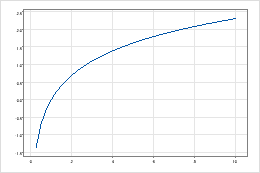# Natural log (log base e) function

To use this function, choose Calc > Calculator.

Calculates logarithms to the base e, where e is the constant equal to approximately 2.71828. The natural log of any positive number, n, is the exponent, x, to which e must be raised so that ex = n. For example, e2 = 7.389, so the natural log of 7.389 is 2.

The following graph represents the natural log function.## Syntax

LN(number)

In number, specify the value or column of values. Minitab calculates the value x such that ex = the number. If you enter 0 or a negative number, Minitab stores a missing value symbol *.

## Example

Calculator expression Result
LN(7.38905) 2

## Uses

The function of the natural log has many applications, such as modeling exponential growth in biological populations and in financial theory, and calculating radioactive decay.

In statistics, the natural log can be used to transform data for the following reasons:
• To make moderately skewed data more normally distributed or to achieve constant variance
• To allow data that fall in a curved pattern to be modeled using a straight line (simple linear regression)
• To stabilize variation when estimating standard deviations (Z-MR control chart)
• To analyze variability in response data for experiments that include repeat or replicate measurements (factorial design)

The natural log is also used in the calculation of probability density functions for many distributions.

By using this site you agree to the use of cookies for analytics and personalized content.  Read our policy